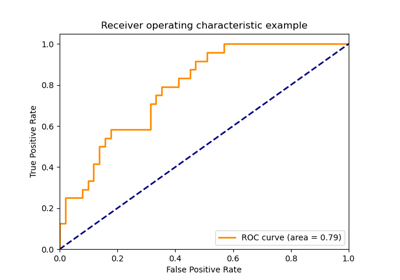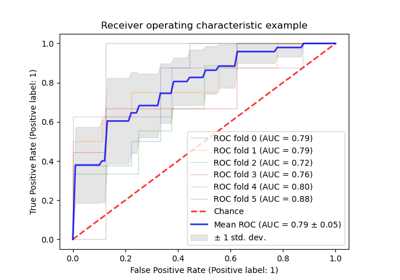# sklearn.metrics.auc¶

sklearn.metrics.auc(x, y)[source]

Compute Area Under the Curve (AUC) using the trapezoidal rule.

This is a general function, given points on a curve. For computing the area under the ROC-curve, see roc_auc_score. For an alternative way to summarize a precision-recall curve, see average_precision_score.

Parameters:
xndarray of shape (n,)

X coordinates. These must be either monotonic increasing or monotonic decreasing.

yndarray of shape, (n,)

Y coordinates.

Returns:
aucfloat

Area Under the Curve.

roc_auc_score

Compute the area under the ROC curve.

average_precision_score

Compute average precision from prediction scores.

precision_recall_curve

Compute precision-recall pairs for different probability thresholds.

Examples

>>> import numpy as np
>>> from sklearn import metrics
>>> y = np.array([1, 1, 2, 2])
>>> pred = np.array([0.1, 0.4, 0.35, 0.8])
>>> fpr, tpr, thresholds = metrics.roc_curve(y, pred, pos_label=2)
>>> metrics.auc(fpr, tpr)
0.75


## Examples using sklearn.metrics.auc¶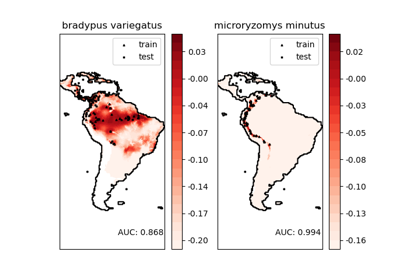Species distribution modeling

Species distribution modeling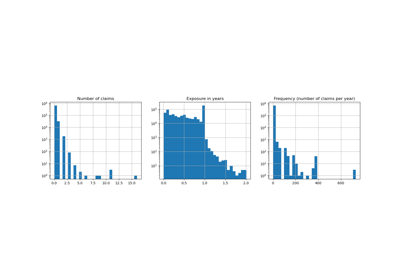Poisson regression and non-normal loss

Poisson regression and non-normal loss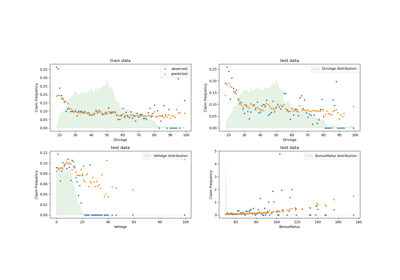Tweedie regression on insurance claims

Tweedie regression on insurance claims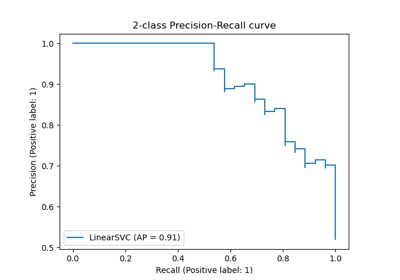Precision-Recall

Precision-Recall